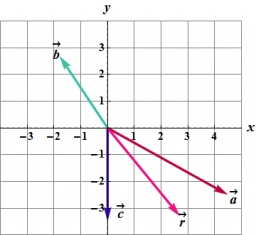# Perpendicular 28823

Points A(1,2), B(4,-2) and C(3,-2) are given. Find the parametric equations of the line that:

a) It passes through point C and is parallel to the line AB,
b) It passes through point C and is perpendicular to line AB.

Result

p1 = (Correct answer is: 1)p2 = (Correct answer is: 1)### Step-by-step explanation:Did you find an error or inaccuracy? Feel free to write us. Thank you!

Tips for related online calculators
Line slope calculator is helpful for basic calculations in analytic geometry. The coordinates of two points in the plane calculate slope, normal and parametric line equation(s), slope, directional angle, direction vector, the length of the segment, intersections of the coordinate axes, etc.
Our vector sum calculator can add two vectors given by their magnitudes and by included angle.